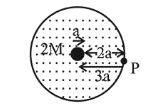# A solid sphere of mass ' M ' and radius 'a'Question:

A solid sphere of mass ' $M$ ' and radius 'a' is surrounded by a uniform concentric spherical shell of thickness $2 \mathrm{a}$ and mass $2 \mathrm{M}$. The gravitational field at distance '3a' from the centre will be:

1. (1) $\frac{2 \mathrm{GM}}{9 a^{2}}$

2. (2) $\frac{\mathrm{GM}}{9 a^{2}}$

3. (3) $\frac{\mathrm{GM}}{3 a^{2}}$

4. (4) $\frac{2 \mathrm{GM}}{3 a^{2}}$

Correct Option: , 3

Solution:

(3) $E_{P}=\frac{G M}{(3 a)^{2}}+\frac{G(2 M)}{(3 a)^{2}}=\frac{G M}{3 a^{2}}$For a part on the surface of a spherical uniform charge

distribution the whole mass acts as a point mass kept at the centre.